# Period of a function

(diff) ← Older revision | Latest revision (diff) | Newer revision → (diff)A number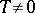such that for any(or) the numbers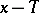andalso belong toand such that the following equality holds: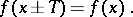The numbers, whereis a natural number, are also periods of. For a functionon an axis or on a plane, any numberis a period; for the Dirichlet functionany rational numberis a period. If a functionhas period, then the function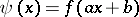, whereandare constants and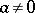, has period. If a real-valued functionof a real argument is periodic on(and is not identically equal to a constant), then it has a least period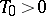and any other real period is a multiple of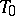. There exist non-constant functions of a complex argument having two non-multiple periods with imaginary quotient, such as for example an elliptic function.

Similarly one defines the period of a function defined on an Abelian group.